# Why error message #NAME? occurs and how to solve it

Uploaded time: March 14, 2022 Difficulty Beginner

A free Office suite fully compatible with Microsoft Office

A free Office suite fully compatible with Microsoft Office

Why error message #NAME? occurs and how to solve it

# Why error message #NAME? occurs and how to solve it

When processing data in a table, the #NAME? error might occur.

There are four possible causes: first, the formula name was misspelled. Second, the double quotation marks were omitted for text values. Third, the colon was omitted in a range reference. Fourth, the formula refers to a name that has not been defined.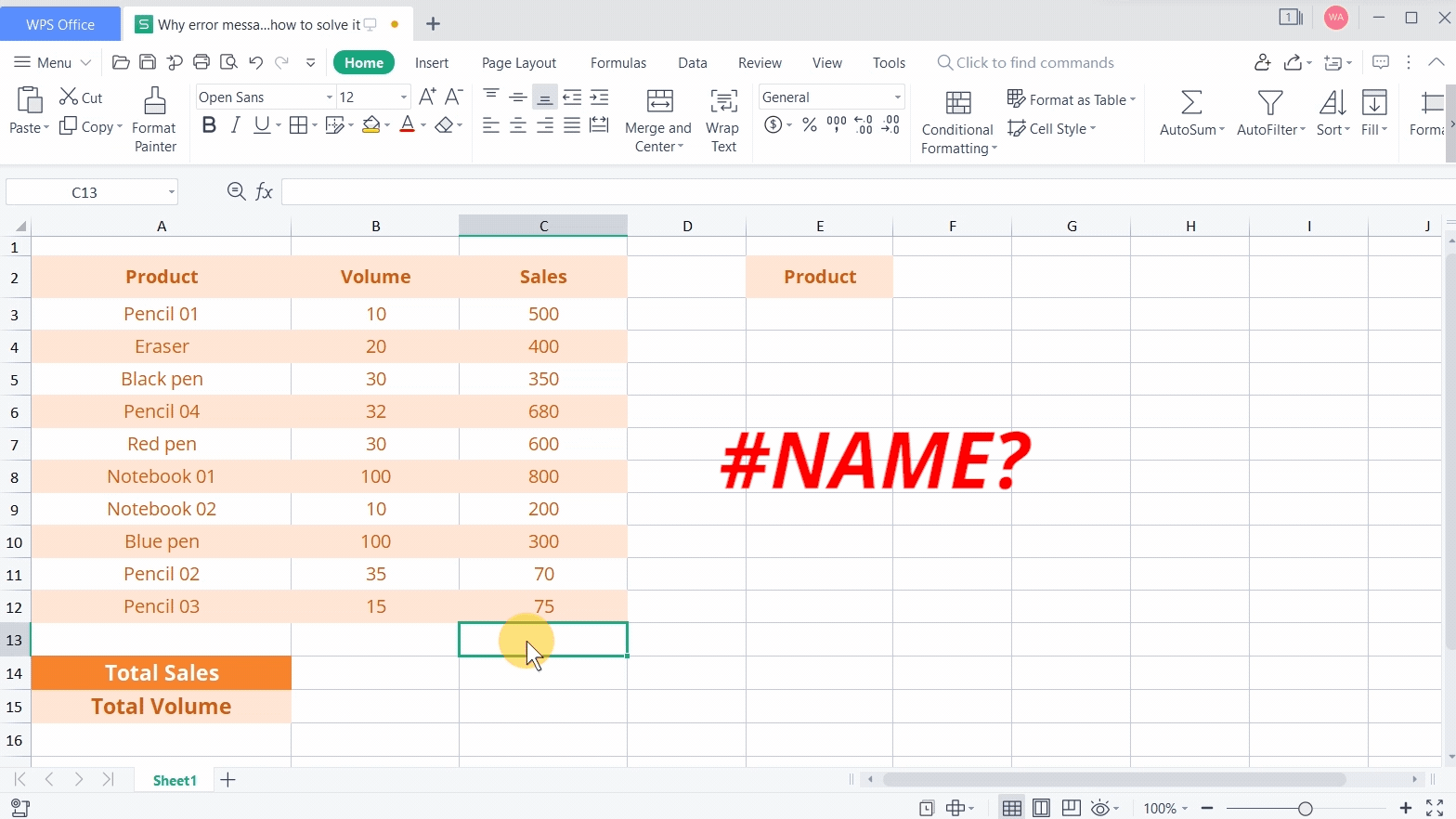First, the formula name was misspelled

Take this table as an example. When we use the formula =SUN(C3:C12) to calculate the total sales of all products, the calculation result will return the error #NAME? This is because we entered the wrong formula name.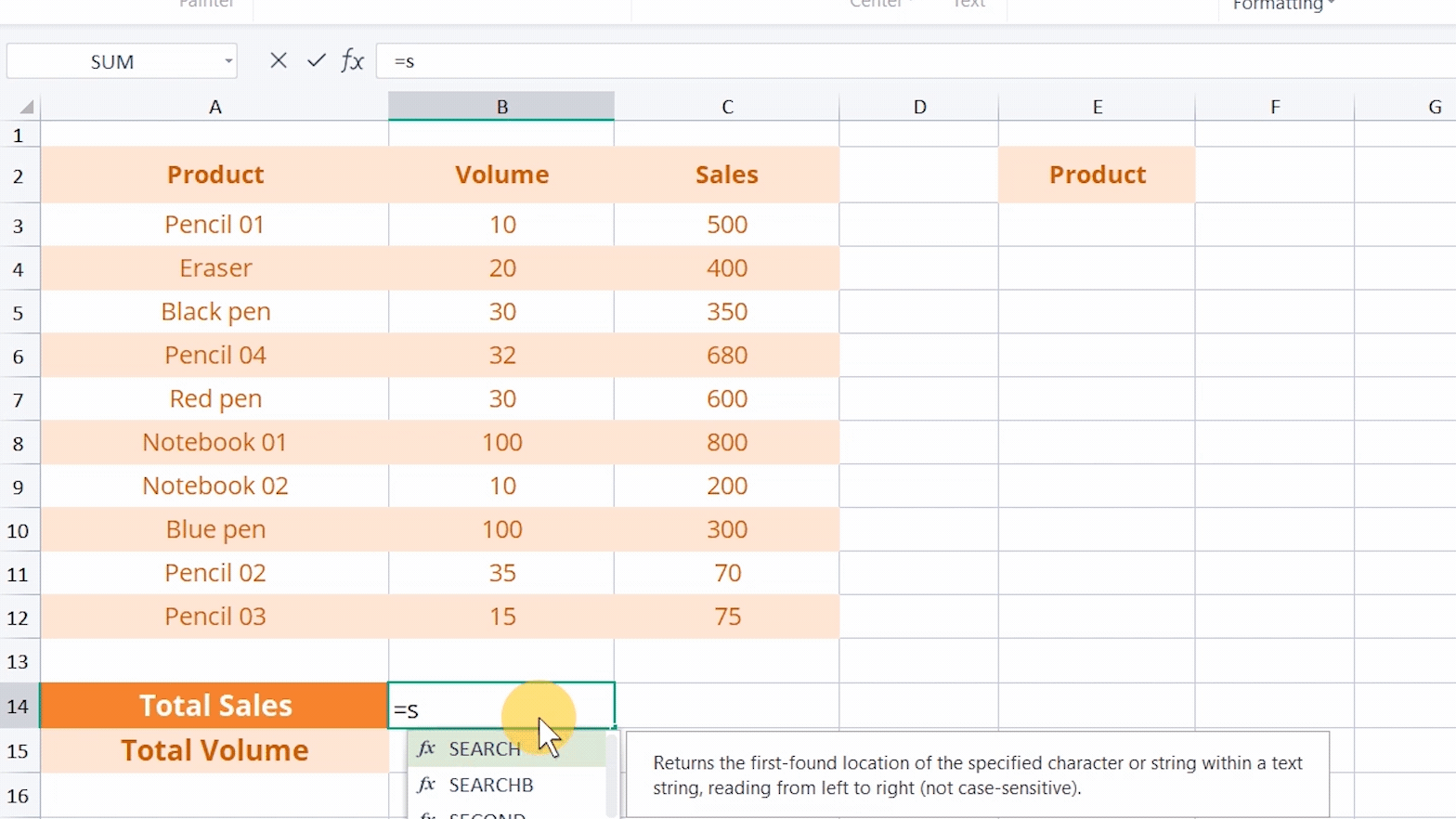To solve this, we need to double-check the spelling of the formula and re-enter the correct name. The correct formula should be SUM so that we can achieve the right calculation result.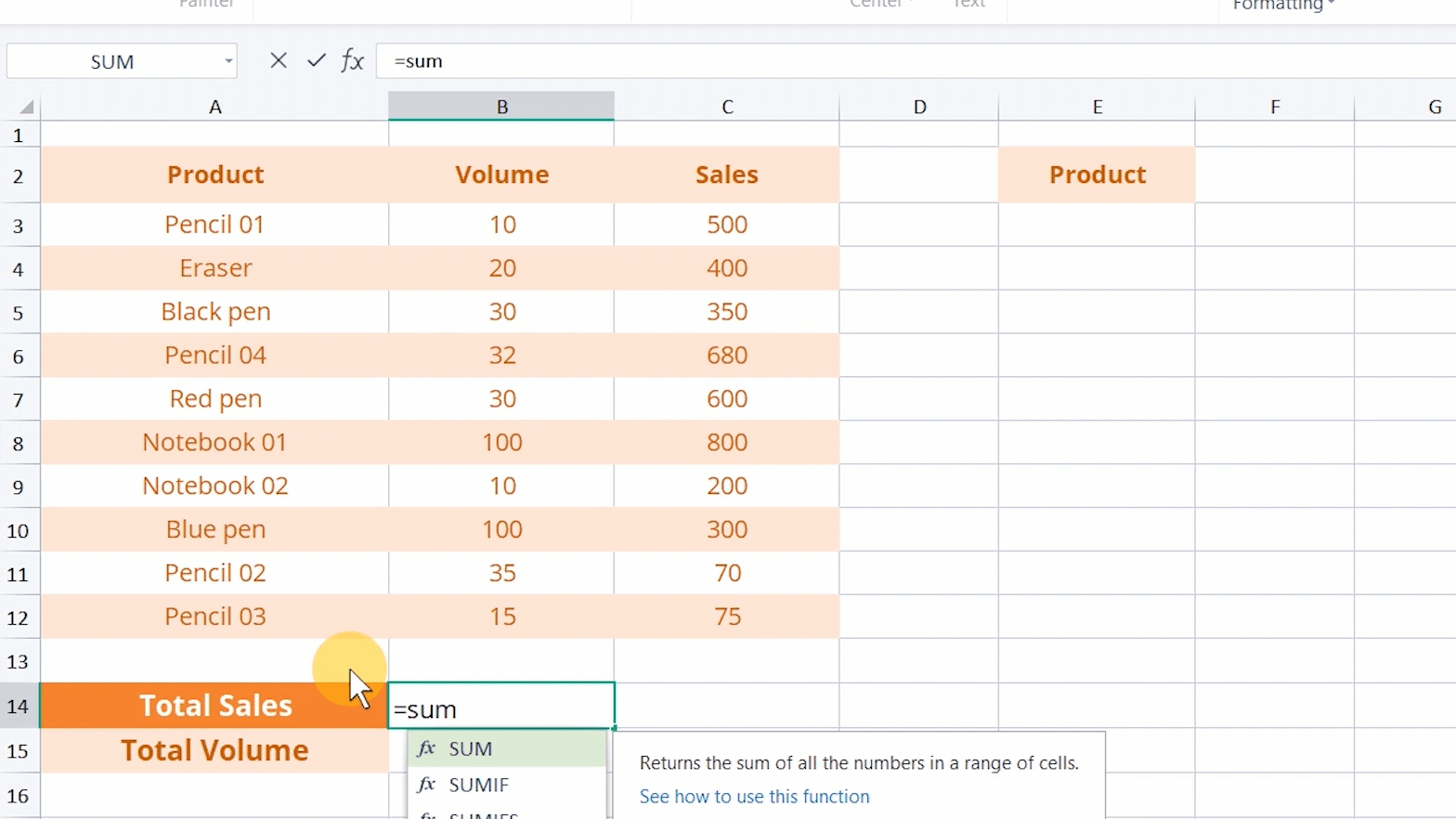Second, the double quotation marks were omitted for text values

Take this table as an example. When we want to replace Pencil with Automatic pencil in the table, enter the formula = substitute (A3, Pencil, Automatic pencil) in cell E3.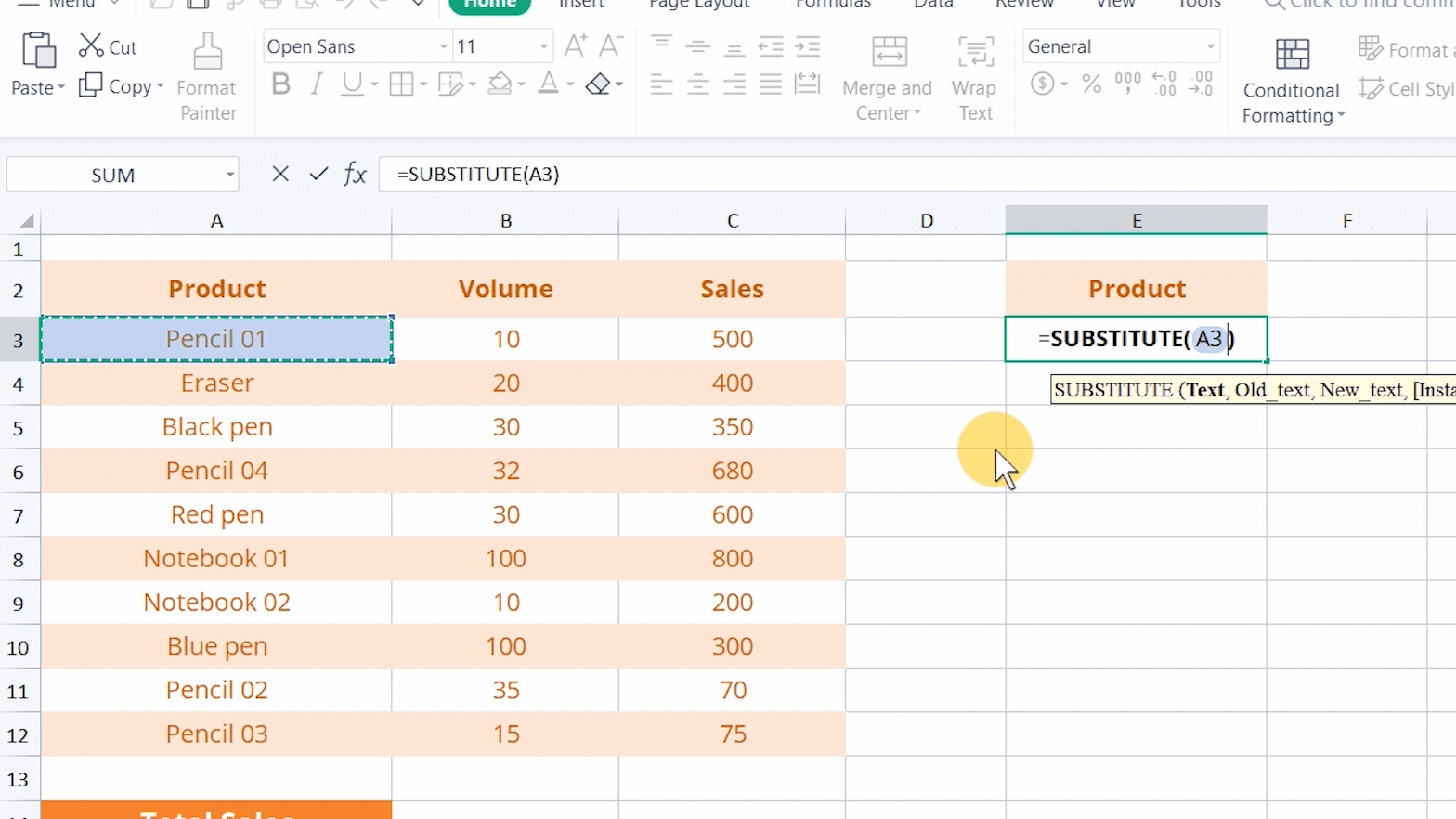The first parameter A3 involves text values. The second parameter is the old text Pencil to be changed, and the third parameter is the new text Automatic pencil to replace the old one. Why does the error value #NAME? appear? .

This is because the text reference misses a double quote.

To solve this, we need to add double quotes to these two text values. After filling in the remaining cells, all Pencil are replaced by Automatic pencil.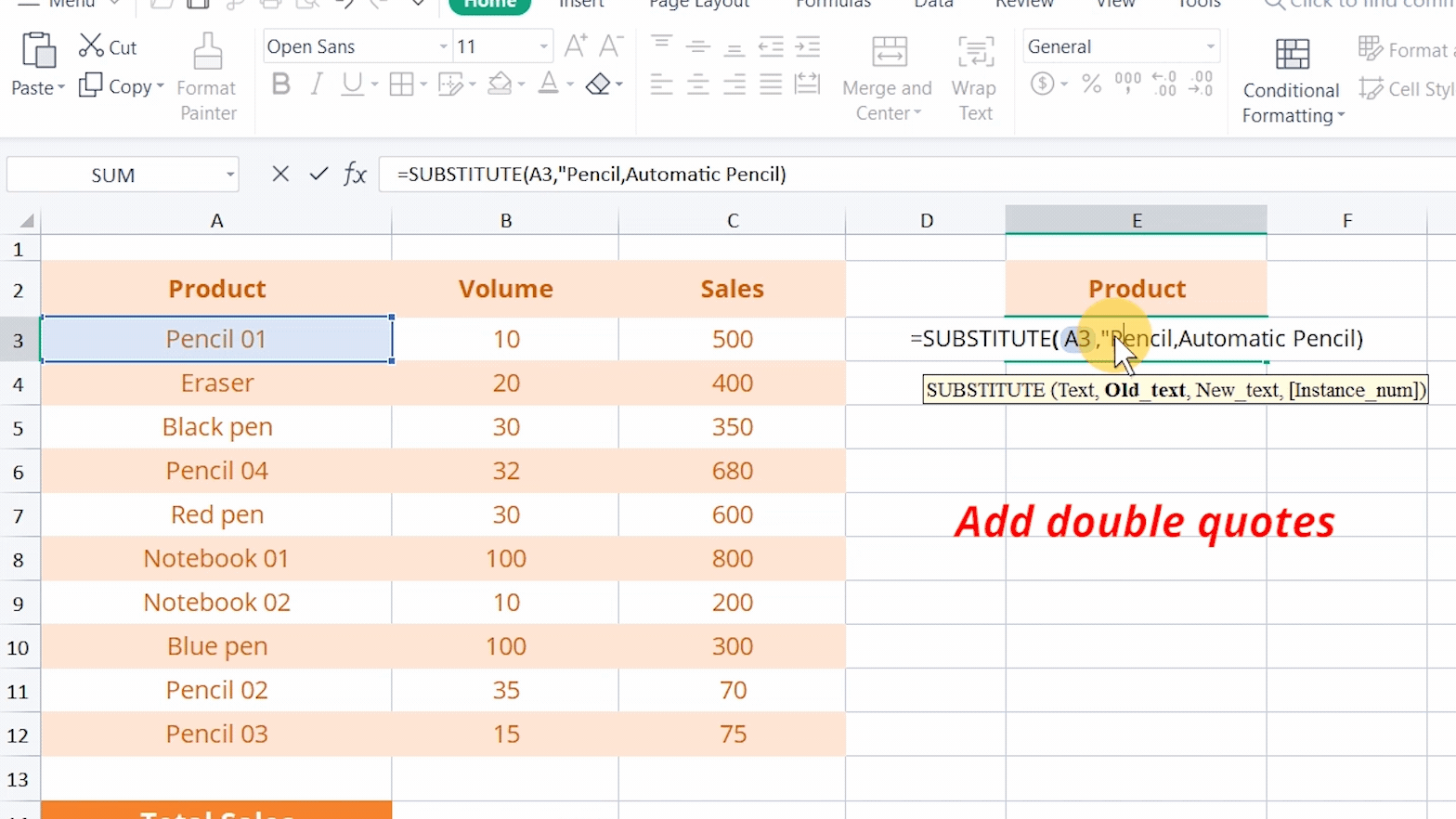Third, the colon was omitted in a range reference.

Take this table as an example. When we want to calculate the total volume of all products, enter the formula  =SUM(B3B12), and the error #NAME? will come to us. This is because no colon is added to the range reference in the formula.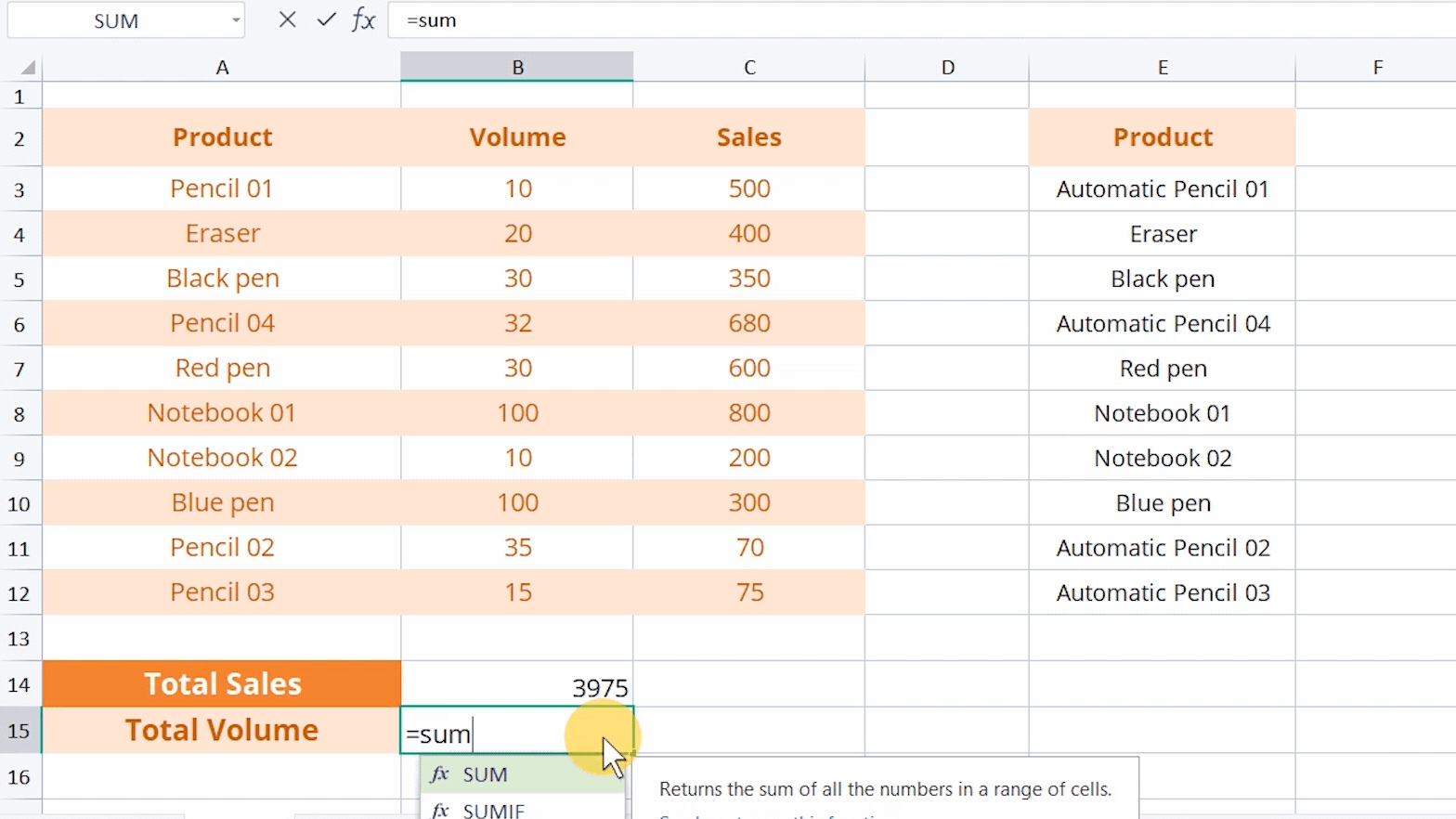To solve this, we only need to add a colon to the range reference.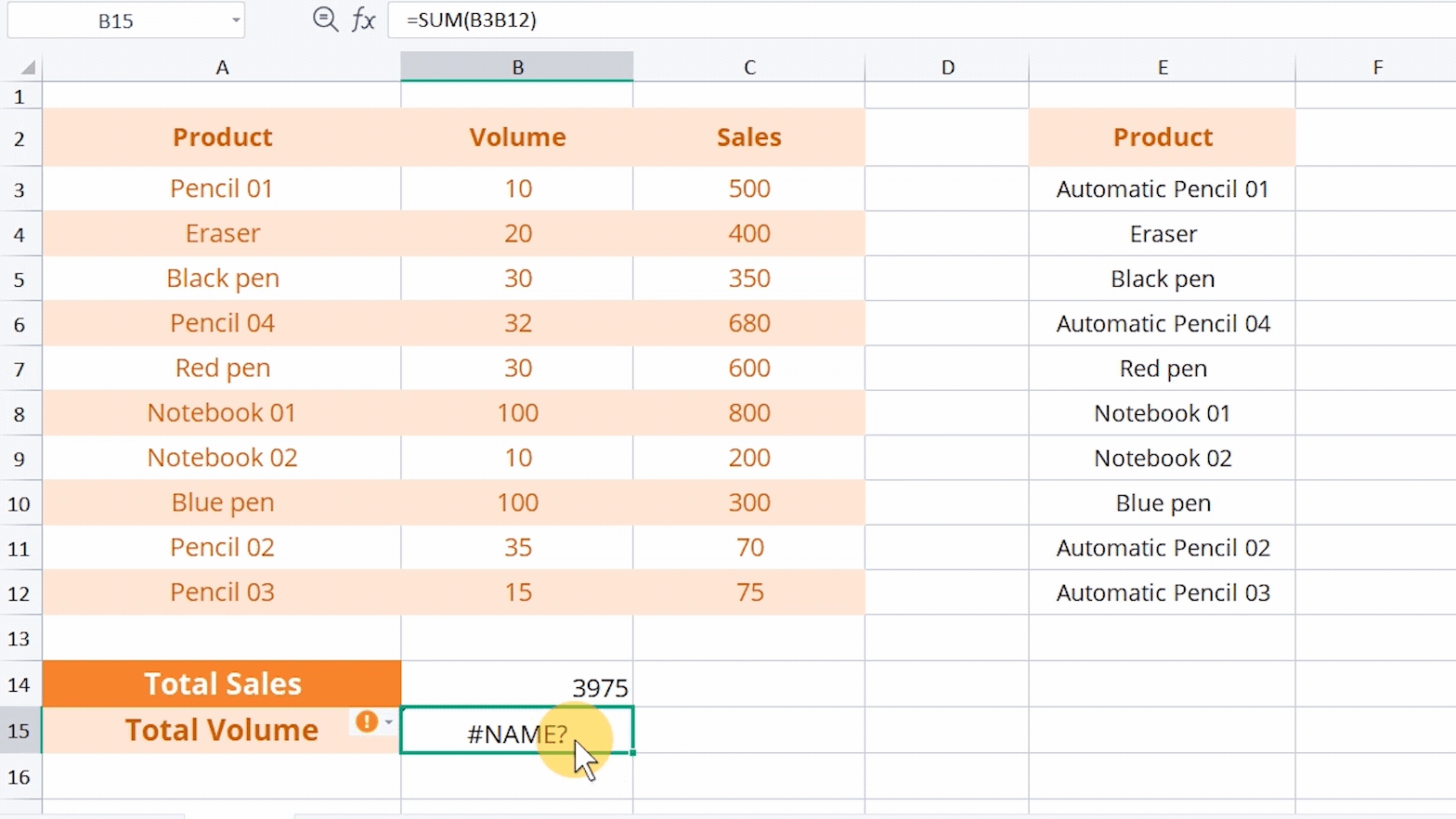Fourth, the formula refers to a name that has not been defined.

When using a formula to calculate data, Name Manager can be used to name the selected area, making the following calculations easier.

For example, we can name the sales volume of products when we want to calculate the total volume.

Click Name Manager in the Formulas tab. Click New in the pop-up dialog, and then name the cell range B3:B12 as Volume.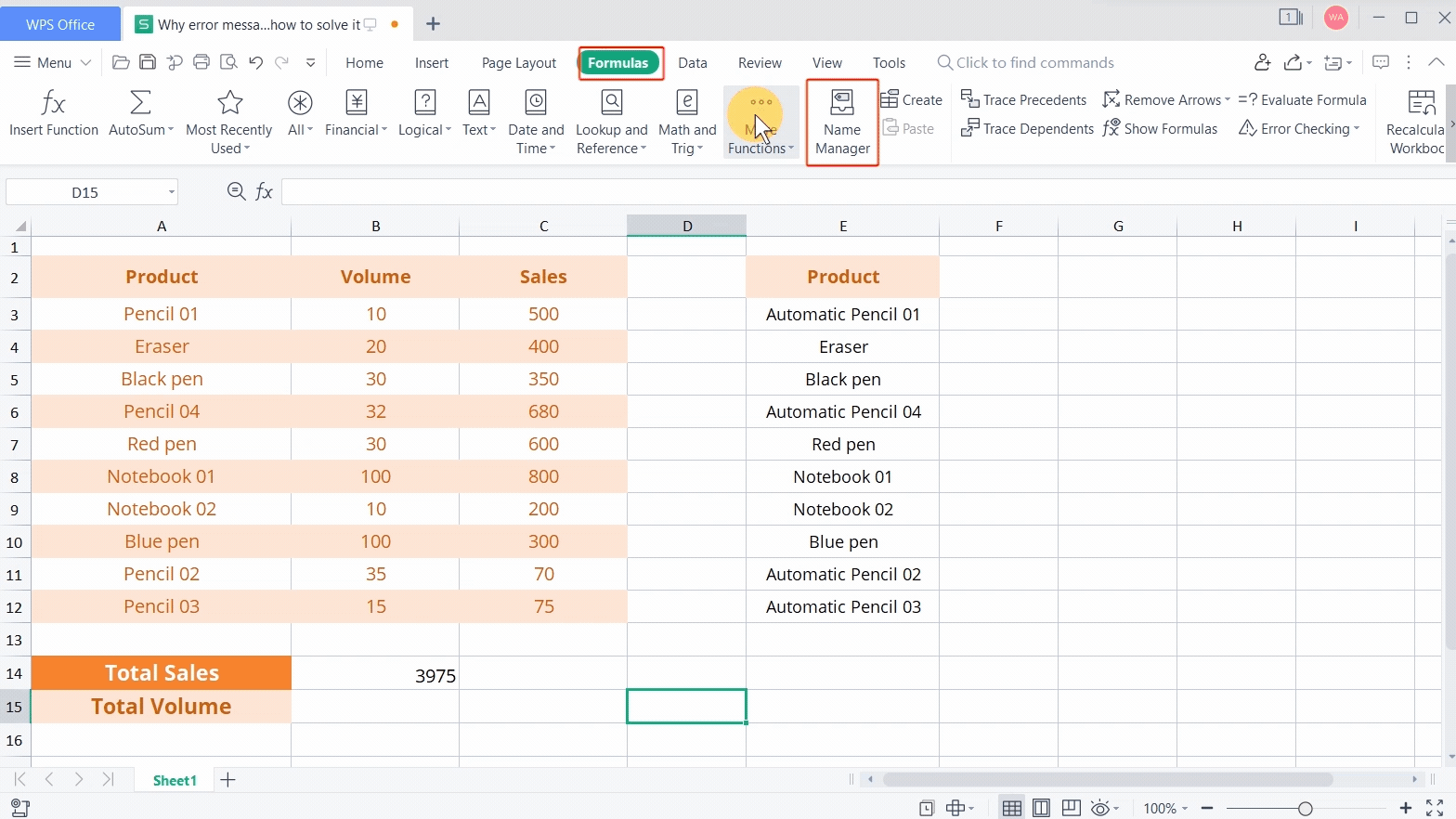At this time, when we use the SUM function to calculate the total volume, we can directly enter the formula =SUM (Volume) to get the result.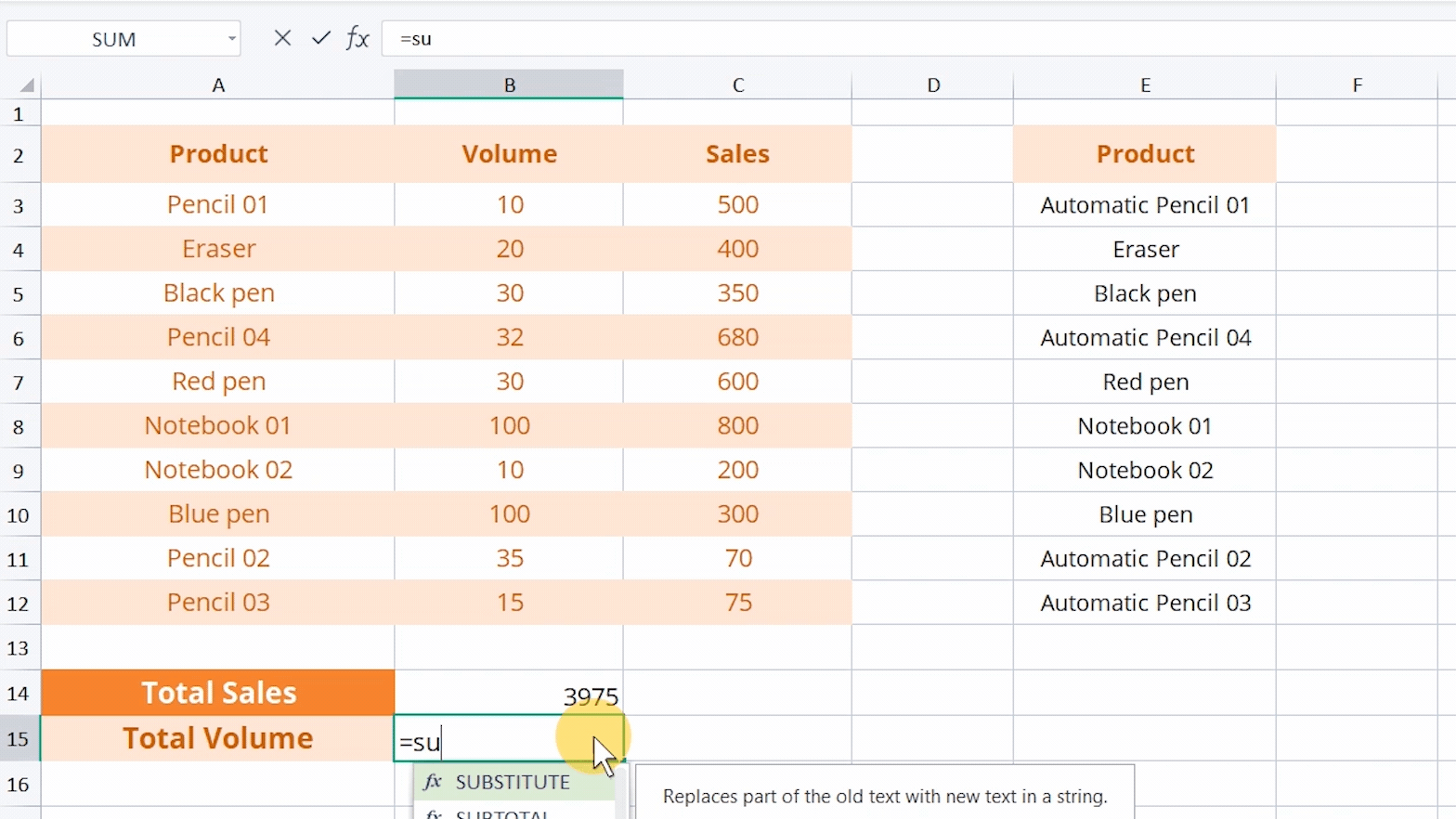When we enter the wrong undefined name, it will display #NAME? At this time, we need to check whether the name is misspelled or we have to add a new custom name.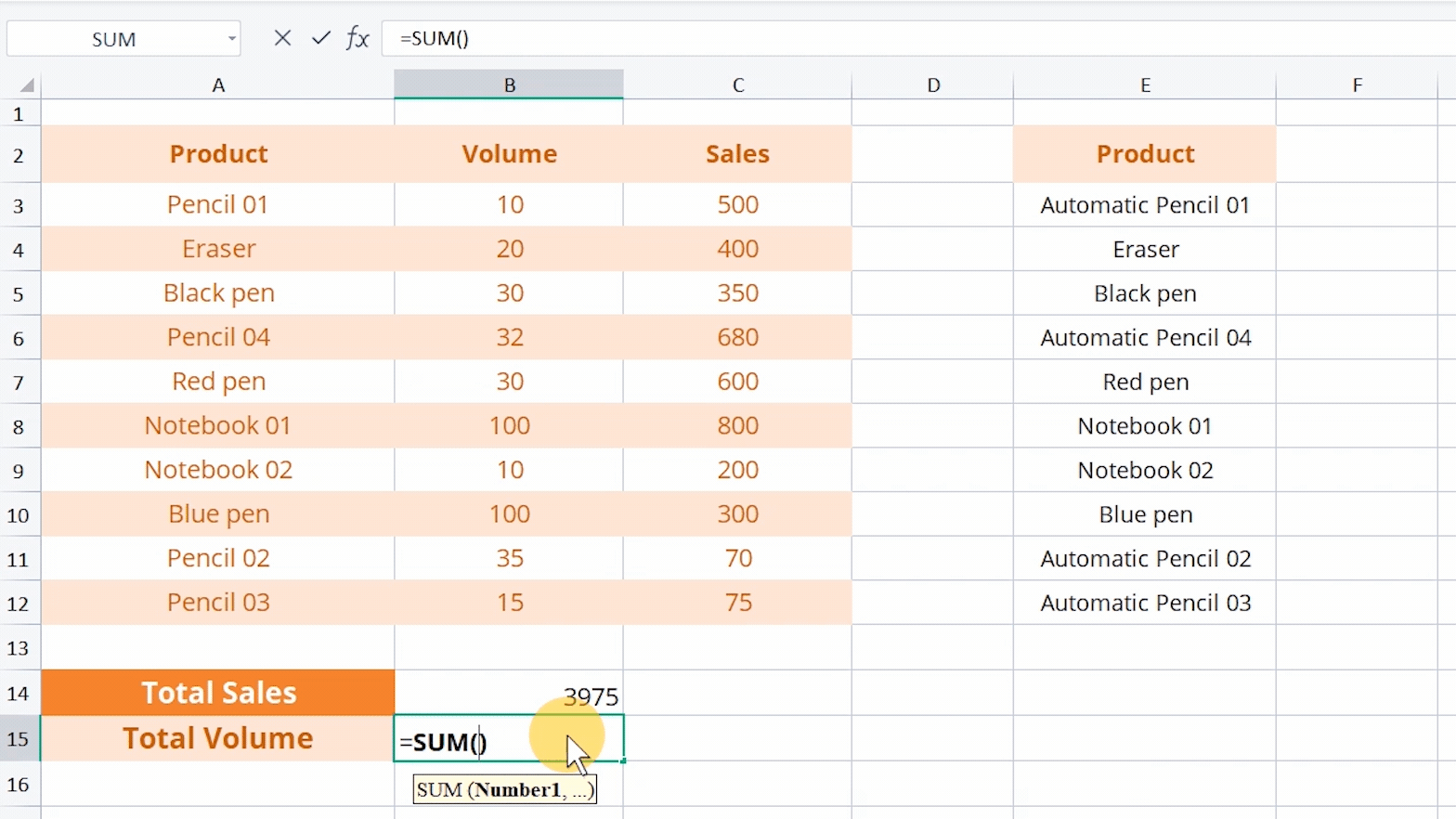WPS Spreadsheet is a powerful chart and form processor. In WPS Academy, you can learn not only how to use Spreadsheet to sort out data but also how to corporate numeric information into graphs. By watching the step-by-step tutorials on WPS Academy, you will become a spreadsheet expert soon.